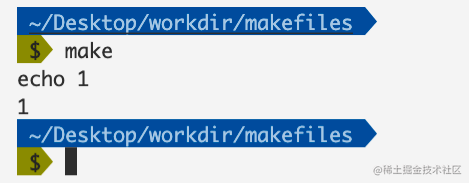# 彻底掌握Makefile（二）## Makefile中的条件判断

### ifeq 和 ifneq

``````cc=g++

main: demo.c
echo \$(cc)

ifeq (\$(cc), gcc)
echo \$(cc) = 相等的语句执行了
else
echo \$(cc) != 不相等的语句执行了
endif

``````cc=g++

main: demo.c
echo \$(cc)

ifneq (\$(cc), gcc)
echo \$(cc) != gcc
else
echo \$(cc) = gcc
endif

``````ifeq (<arg1>, <arg2>)
ifeq '<arg1>' '<arg2>'
ifeq "<arg1>" "<arg2>"
ifeq "<arg1>" '<arg2>'
ifeq '<arg1>' "<arg2>"

ifneq (<arg1>, <arg2>)
ifneq '<arg1>' '<arg2>'
ifneq "<arg1>" "<arg2>"
ifneq "<arg1>" '<arg2>'
ifneq '<arg1>' "<arg2>"

### ifdef 和 ifndef

`ifdef``ifndef`用于判断表达式是否定义过，或者是否为空：

``````foo = 1
main: demo.c
echo demo
ifdef foo
echo define foo
else
echo not define foo
endif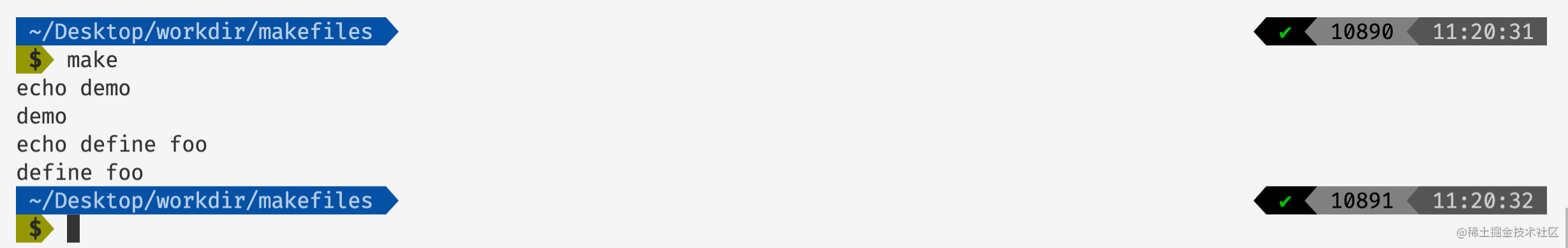``````main: demo.c
echo demo
ifdef foo
echo define foo
else
echo not define foo
endif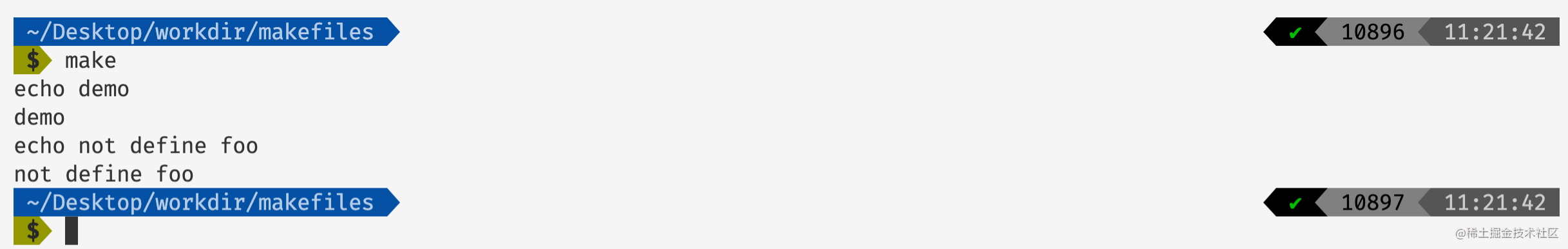``````foo =
main: demo.c
echo demo
ifdef foo
echo define foo
else
echo not define foo
endif

``````bar =
foo = \$(bar)
main: demo.c
echo demo
ifdef foo
echo define foo
else
echo not define foo
endif

## Makfile中的函数

``````\$(<函数名> <函数参数>)

### 字符串函数

#### subst

``````\$(subst <from>,<to>,<text>)

• 字符串替换函数。
• 表示文本，这个函数会将text当中是的字符串替换为。
``````s = ii am learning makefile
ss = \$(subst ii, you, \$(s))

main: demo.c
echo demo
echo \$(s)
echo \$(ss)

#### patsubst

``````\$(patsubst <pattern>,<replacement>,<text>)

• pattern 表示第一个参数，用于表示如何对 text 进行匹配。
• replacement 表示第二个参数 表示如何对匹配的字符进行重写。
• patsubst在进行匹配替换的时候，会先将字符串text根据空格或者tab键或者回车换行符进行分割，然后一一的进行替换和匹配。

``````s = a.c b.c d.c abc.c abo.c
ss = \$(patsubst %.c, %.o, \$(s))

main: demo.c
echo demo
echo \$(s)
echo \$(ss)

• 在上面的makefile当中首先会将\$(s)当中的字符串按照回车换行、空格以及tab键将\$(s)当中的进行分割，然后一个一个进行patsubst操作，然后将得到结果合并起来。

• 在上面的patsubst函数当中%符号是一个通配符，他匹配了.c前面的所有符号。

• 在pattern当中的符号为"%.c"，这个表示用%匹配了.c前面的所有的字符。

• 在replacement当中的字符串为"%.o"，这个%用于表示在pattern当中匹配到的字符串，比如对于abo.c来说%就代表了abo，因此被替换的字符串就是abo.o。

#### Strip

``````\$(strip <string>)

• 主要功能去掉字符串 string 首尾的空格。

#### findstring

``````\$(findstring <find>,<text>)

• 这个函数的作用是从字符串当中寻找字符串，如果找到了字符串就返回字符串，否则返回空。

filter

``````\$(filter <pattern...>,<text>)

``````s = a.c abo.c s.o s.y x.o x.y
ss = \$(filter %.c %.o, \$(s))

main: demo.c
echo \$(ss)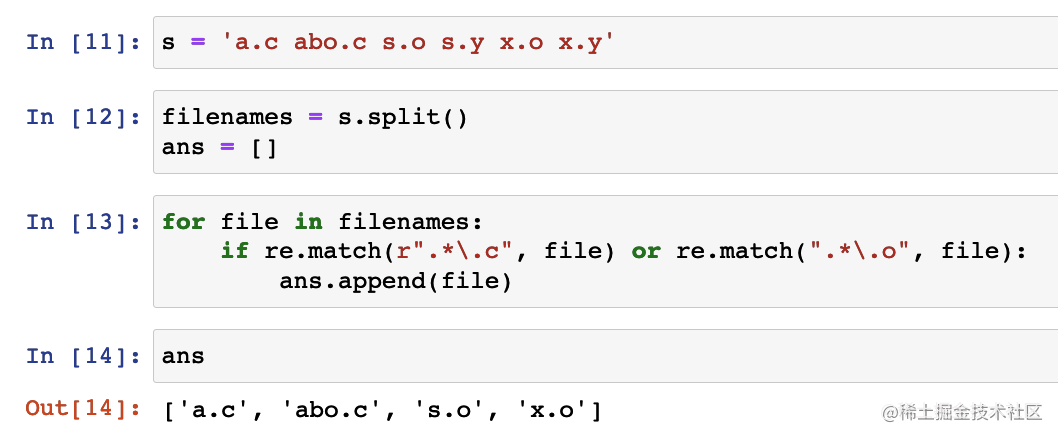filter-out

``````s = a.c abo.c s.o s.y x.o x.y
ss = \$(filter-out %.c %.o, \$(s))

main: demo.c
echo \$(ss)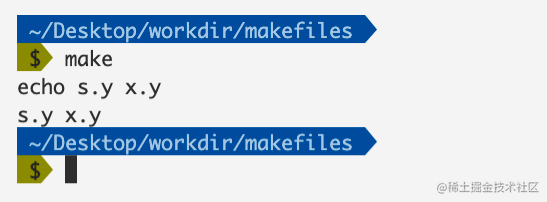#### sort

``````s = g a b c d e f  a a a a
ss = \$(sort \$(s))

main: demo.c
echo \$(ss)

#### word

``````\$(word <n>,<text>)

``````s = g a b c d e f  a a a a
ss = \$(word 1, \$(s)) # 取出第一个字符

main: demo.c
echo \$(ss)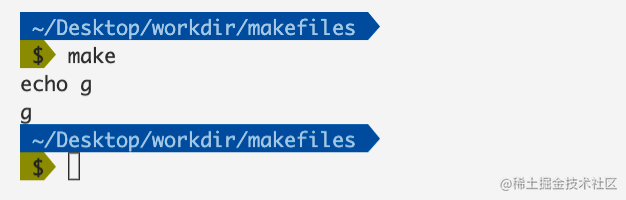#### wordlist

``````\$(wordlist <start>,<end>,<text>)

``````s = g a b c d e f  a a a a
ss = \$(wordlist 1, 5, \$(s))

main: demo.c
echo \$(ss)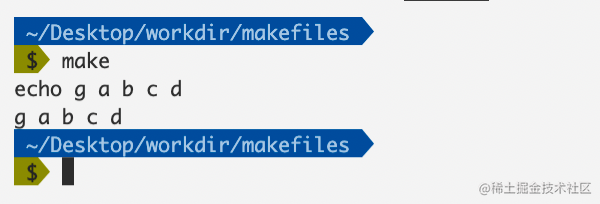#### words

``````s = 1 2 3 4 5
ss = \$(words \$(s))

main: demo.c
echo \$(ss)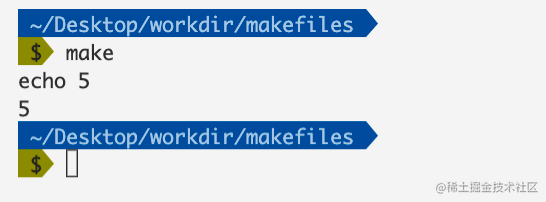#### firstword

``````s = 1 2 3 4 5
ss = \$(firstword \$(s))

main: demo.c
echo \$(ss)